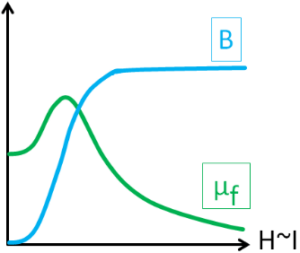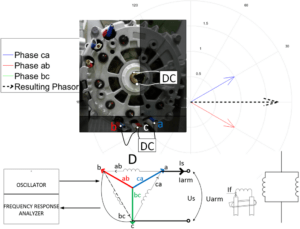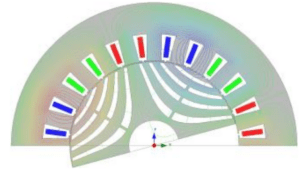# Tag: Matlab### Obtaining the Inductance with Dependence on Frequency and Amplitude of the Applied Alternating Current via Measurements and Validation of Considered Non-linearity and Saturation Effects with Lumped Parameter Model

This publication provides the process for obtaining of a self-inductance of electrical machines with a dependence on frequency and amplitude of an applied alternating current and consideration of a non-linearity and saturation effects in the equivalent circuit lumped parameter model that would have the same frequency and time domain characteristic as some desired investigated electrical machine. The values of inductances were calculated from measured impedances for wide ranges of frequencies and currents according to the theory of a complex inductance and iron losses. The measured data were analyzed and summarized in a table and then used for modeling of a automotive alternator. The model was supplied with curve fitted data. The simulation of a common automotive test case was obtained and compared with measurements. Good match between the theory and measurements and reasonability of the suggested approach were confirmed.

This content is for Basic and Premium members only.### Standstill Frequency Response Test for Obtaining Parameters of Six Phase Double Delta Salient-Pole Synchronous Machine on Example of Claw-Pole Alternator

The current publication introduces an approach for obtaining parameters of a six phase double delta salient-pole synchronous machine, based on the standstill frequency response test. The described approach was verified by measurements on a automotive claw-pole alternator, done in a laboratory on a test bench.

This content is for Basic and Premium members only.